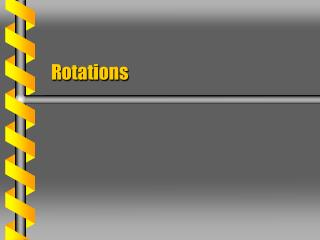# Rotations - PowerPoint PPT PresentationDownload PresentationRotations

RotationsDownload Presentation## Rotations

- - - - - - - - - - - - - - - - - - - - - - - - - - - E N D - - - - - - - - - - - - - - - - - - - - - - - - - - -
##### Presentation Transcript

1. Rotations

2. Space coordinates are an inertial system. Fixed in space Body coordinates are a non-inertial system. Move with rigid body Space and Body x3 x3 x2 x2 x1 x1

3. A linear transformation connects the two coordinate systems. The rotation can be expressed as a matrix. Use matrix operations Distance must be preserved. Matrix is orthogonal Product is symmetric Must have three free parameters Matrix Form x3 x2 x1

4. Axis of Rotation • An orthogonal 3 x 3 matrix will have one real eigenvalue. • Real parameters • Cubic equation in s • The eigenvalue is unity. • Matrix leaves length unchanged • The eigenvector is the axis of rotation. x3 x2 x1 +1 for right handedness

5. The eigenvector equation gives the axis of rotation. Eigenvalue = 1 The trace of the rotation matrix is related to the angle. Angle of rotation c Trace independent of coordinate system Single Rotation

6. Rotating Vector • A fixed point on a rotating body is associated with a fixed vector. • Vector z is a displacement • Fixed in the body system • Differentiate to find the rotated vector. x3 x2 x1

7. The velocity vector can be found from the rotation. The matrix W is related to the time derivative of the rotation. Antisymmetric matrix Equivalent to angular velocity vector Angular Velocity Matrix

8. The terms in the W matrix correspond to the components of the angular velocity vector. The angular velocity is related to the S matrix. Matching Terms

9. The angular velocity can also be expressed in the body frame. Body version of matrix Body Rotation x3 x2 x1